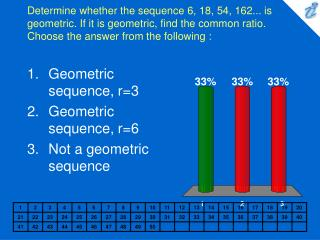DownloadDownload PresentationDetermine whether the sequence 6, 18, 54, 162... is geometric. If it is geometric, find the common ratio. Choose the ans

# Determine whether the sequence 6, 18, 54, 162... is geometric. If it is geometric, find the common ratio. Choose the ans

Télécharger la présentation## Determine whether the sequence 6, 18, 54, 162... is geometric. If it is geometric, find the common ratio. Choose the ans

- - - - - - - - - - - - - - - - - - - - - - - - - - - E N D - - - - - - - - - - - - - - - - - - - - - - - - - - -
##### Presentation Transcript

1. Determine whether the sequence 6, 18, 54, 162... is geometric. If it is geometric, find the common ratio. Choose the answer from the following : • Geometric sequence, r=3 • Geometric sequence, r=6 • Not a geometric sequence

2. 1. 2. 3. Determine the 5th term of the geometric sequence {image} Choose the answer from the following : • {image} • {image} • {image}

3. 1. 2. 3. The common ratio in a geometric sequence is {image} , and the fourth term is {image} . Find the third term. Choose the answer from the following : • {image} • {image} • {image}

4. Find the sum. 1 + 4 + 16 + ... + 16,384. Choose the answer from the following : • 21,845 • 5,461 • 87,381

5. 1. 2. 3. Find the sum of the infinite geometric series. {image} Choose the answer from the following : • {image} • {image} • {image}